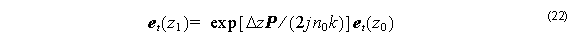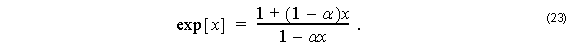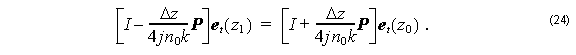Formally, the solution to the BPM equations (whether Full-Vector, Semi-Vector, or Scalar) iswhere Δz1z0 . If the field et  is assumed known at a transverse plane z0 , then the above equation will calculate the field at some other plane z1 . A rational function is needed to approximate the exponent of the operator. One of the simplest expressions is the followingThis is a rational approximation to the exponent. If α = 0.5, it is called the Padé(1,1) approximant. It is accurate for small values of the argument, (values much less than 1). This is one of the limitations to the size of the propagation step, Δz . Sometimes higher order Padé approximants are used to permit larger propagation steps, or to accommodate rapid variations from high index contrast or light propagating at a wide angle from the optical axis.

In the case α = 0.5, Equation 23 generates the first three terms of the MacLaurin series expansion for exp [ x ] , and its application leads to the Crank-Nicholson method,In OptiBPM, the operator P is a large sparse matrix that approximates the partial derivatives as finite differences. In the above equation, the field is assumed known at  z =  z0 , and is to be found on the plane z z1 . The operator P is applied to the unknowns to be found in the vector et ( z1 ) . Since Equation 24 does not give the unknown et ( z1 )  directly, but instead only as a solution to an equation, the resulting numerical method is called ‘implicit’. To progress from one transverse plane to the next using an implicit method requires the solution of the above set of linear equations.

The variable α is called the Scheme Parameter, the usual value is 0.5, partly because it is the most accurate representation of the exponent, but also because this often realizes the most stable method. In Equation 24, it is possible to show (before the introduction of lossy boundary conditions) that the norm of the operator on the left is the same as the operator on the right. Therefore the application of many steps should lead to no change in the norm of the solution vector  et. This guarantees the stability of the method, at least in the sense of conservation of energy.